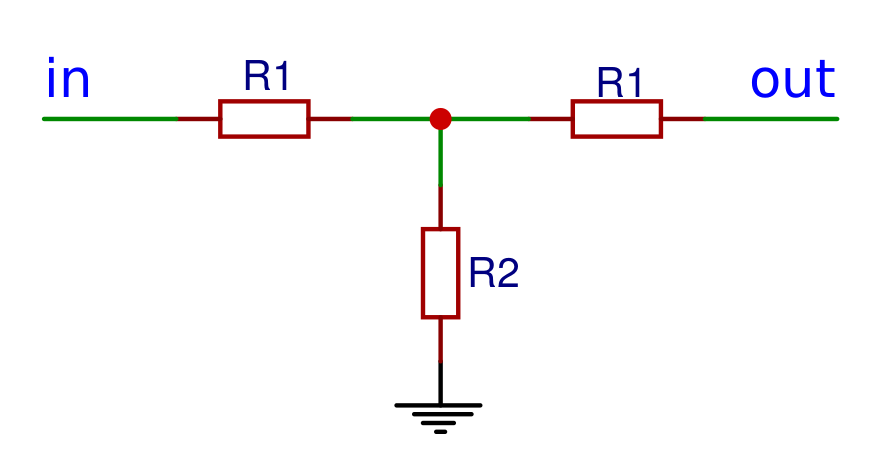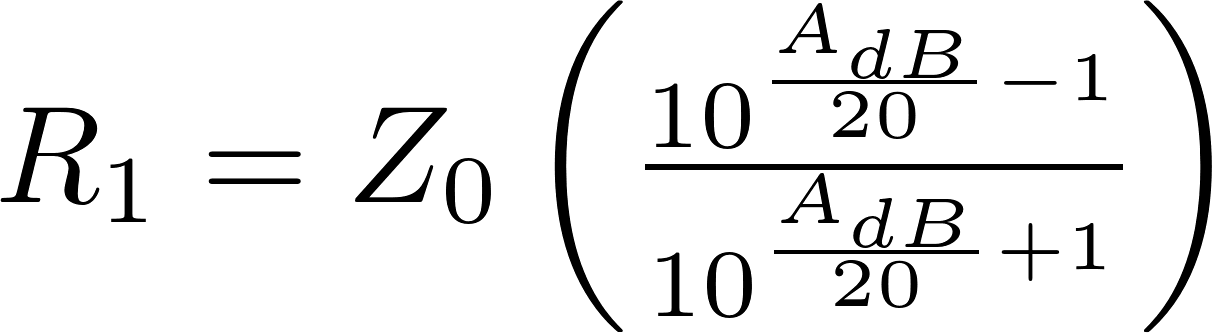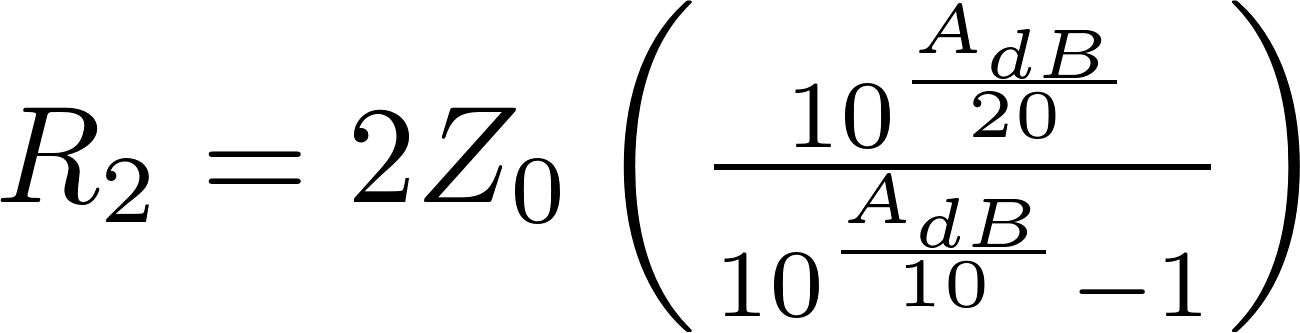# T attenuator

The T attenuator is a type of attenuator that looks like the letter T. The T attenuator consists of three resistors. Two of these are connected in series and the other one is connected from between the two other resistors to ground. The resistors in series often have the same resistance.### Formulas

The following formulas can be used to calculate the resistance of all resistors for a given attenuation and impedance.### Calculator

Enter the attenuation and impedance to calculate the resistance of the resistors.